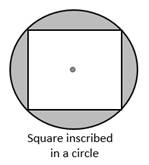Chapter 8.CT, Problem 13CT### Elementary Geometry for College St...

6th Edition
Daniel C. Alexander + 1 other
ISBN: 9781285195698

#### Solutions

Chapter
Section### Elementary Geometry for College St...

6th Edition
Daniel C. Alexander + 1 other
ISBN: 9781285195698
Textbook Problem
1 views

# In the figure, a square is inscribed in a circle. If each sideof the square measures 4 2 in., find an expression for theexact area of the shaded region.                                 _To determine

To find:

An expression for the exact area of the shaded region.

Explanation

Given:

In the fig, a square is inscribed in a circle. If each side of the square measures 42 in.

Theorem:

1. The area A of a circle whose radius has the length r is given by the formula, A=πr2.

2. The area A of the square whose length of the side a is given by A=a2.

From the given, the lengths of the side of the square is 42 in.

Here a=42 and d=a2+a2=2a2=a2, then r=d2=a22=a2

The area of the shaded region = Area of the circle – Area of the square

A=πr2-a2

A=πa22-a2

A=πa22-a2</

### Still sussing out bartleby?

Check out a sample textbook solution.

See a sample solution

#### The Solution to Your Study Problems

Bartleby provides explanations to thousands of textbook problems written by our experts, many with advanced degrees!

Get Started

#### Find more solutions based on key concepts